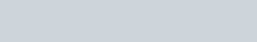In this tutorial I introduce you to how you calculate the median, lower and upper quartiles and percentiles for a continuous random variable.

Finding the Median Quartiles, Percentiles from a pdf or cdf | ExamSolutions - youtube Video

## Example

In this video I show you how to calculate the median and lower quartile from a probability density function (p.d.f) by way of a worked example.

Below is the example which you may like to try first as part of your maths revision and then check your methods with the worked solution.

The random variable X had a p.d.f. given byFind the median m, and the lower quartile Q1.

Median and Quartiles of a continuous random variable. Example | ExamSolutions - youtube Video